# How does a radius gauge work?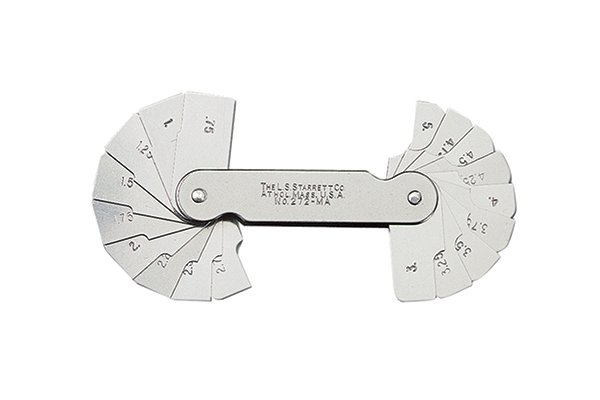A radius gauge works as a concave and convex referencing device.

It is used to clarify how close the radius in question (on a workpiece) compares to the precisely machined radii on the gauge.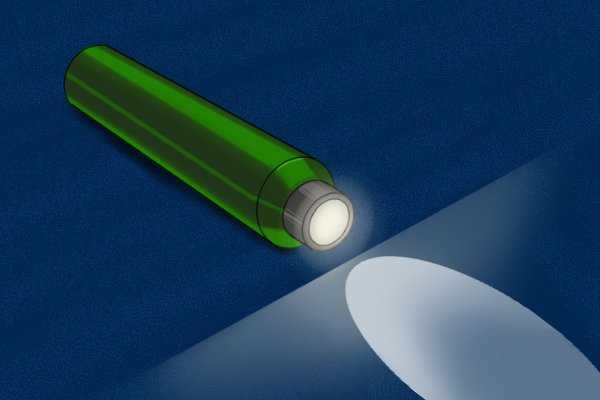A light source should be placed behind the object being measured to help identify any gaps between the surfaces.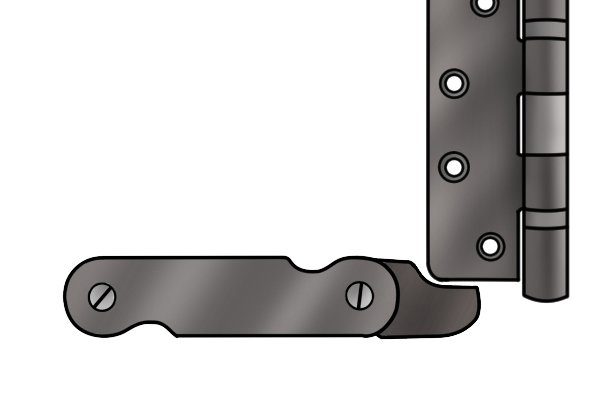The precisely machined radius of the gauge is pressed closely against an object with either a concave or convex radius.

The two distinct measuring processes are described below.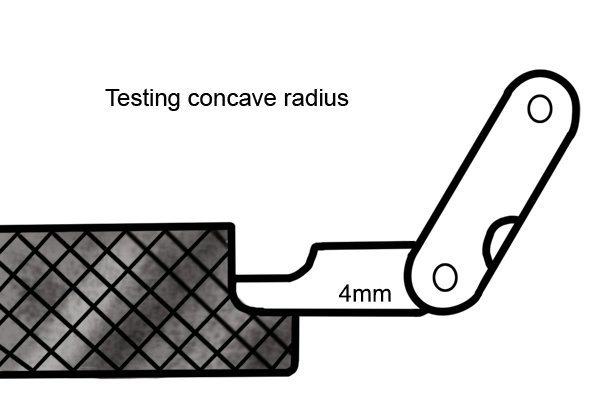A radius gauge can be used to test the size of a concave radius on an existing object.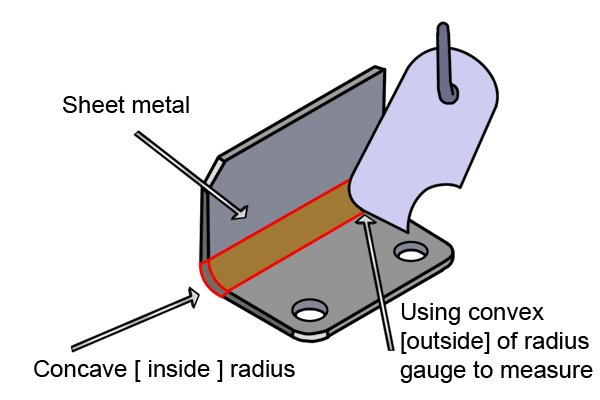Here the external convex radius of the gauge is used to identify the internal concave radius of sheet metal.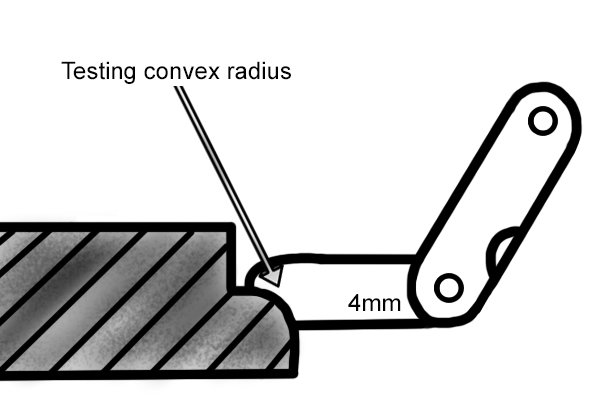A radius gauge can be used to test the size of a convex radius on an existing object.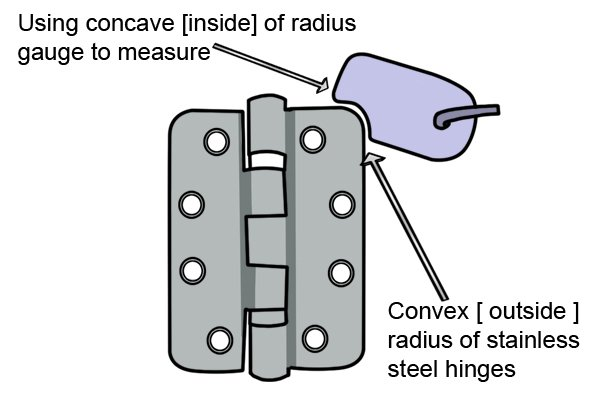Here the internal concave radius of the gauge is used to identify the external convex radius of a hinge.